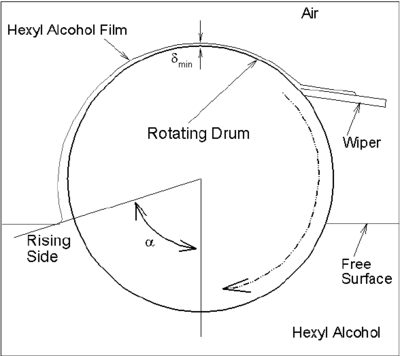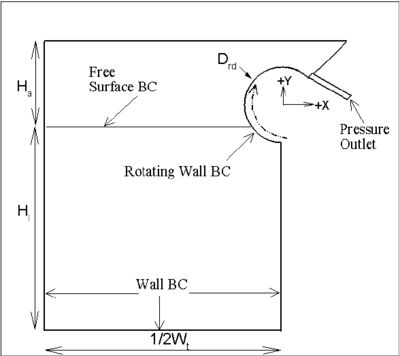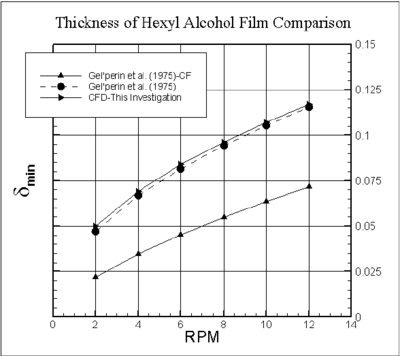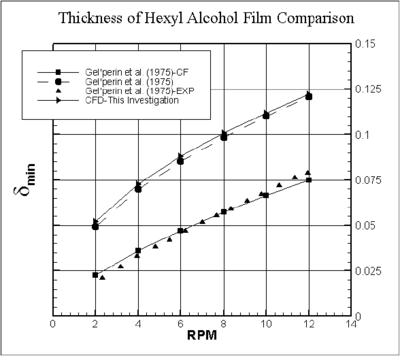## Abstract

In this research, the free surface of micro film of hexyl alcohol on a partially submerged rotating drum has been predicted using CFD (computational fluid dynamics) and compared with experimental data and analytical solution. The trend of has been found very comparable with experimental and analytical solution. A Matlab code was used to model the flow and film dynamics. Computationally it was an unsteady state problem and semi-steady state was achieved. No surfactant was present on the surface. The speed of the moving rotating drum was set a rpm. With the increase of rotating drum, the minimum film thickness increases with rpm (for ${\textstyle \alpha _{o}}$ = 47$^{0}$ and for ${\textstyle \alpha _{o}}$= 58$^{0}$).

Keywords: Liquid film, cfd, vof, moving surface, hexyl alcohol

## 1. Introduction

This paper presents a two-dimensional (not a 3D as performed in  ) computational CFD model describing an unsteady state thin liquid hexyl alcohol film on the RD adjacent to the surface of a partially submerged rotating drum (RD). The liquid film was modeled by volume of fluid, VOF  . A RD submerged in a fluid picks up fluid on the outer surface of the RD (Figure 1). Few parameters e.g. D$_{\mathit {rd,\rho ,\sigma ,\mu ,\alpha _{o}}}$, and rpm influence the free surface as well (refer to list of symbols). These properties form various nondimensional numbers, e.g., Ca, We, Re, Fr (refer to list of symbols) . Solving complete Navier-Stokes equation with VOF as performed here would allow predicting the free surface provided numerical error is minimized. However, VOF has limitation like all other free surface modeling approaches.

Literature review suggests that there is limited focus  [3,4,5,6,7] on this topic and only a few correlations are available based on the experimental or analytical simplification  [8,9,10]. The surface area of the liquid film and the amount of fluid being carried by an RD are important parameters for design purpose (e.g. cooling of final molasses in a sugar factory  [10,11,12] and pharmaceutical drug delivery of solid oral dosage forms film coatings  [13,14,15].Figure 1. Schematic diagram of the thin film on a partially-submerged rotating drum

The dynamics of liquid film on a straight plate   is relatively easy compared to the investigation performed here. Prediction of dynamic contact line   is still under investigation. This paper will particularly focus when a wiper is used to wipe the film of hexyl alcohol. The prime motivation of this investigation is that there is published experimental data for hexyl alcohol which occur at ${\textstyle \alpha }$=180$^{0}$ (Figure 1). There is no accurate measuring technique available to measure the film thickness  , even though, the experimental effort has been lifted. Most of the studies avoided curved moving plane movement  [19,20] in detail.

After the computational domain and model equation, this paper will continue with the computational results compared with the experimental and analytical solution of  [8,9]. This article assumes that there is no foam   formation near RD and no vaporization   on the liquid film.

## 2. Computation Domain

The computational domain of an RD rotating anti-clockwise on a free liquid surface is shown in Figure 2. Various boundary conditions are shown in Table 1.

Table 1. List of BC for the model
 Serial Location Boundary 1 RD wall Moving wall with rpm 2 Top surface of the tank Outflow 3 Walls of the tank Wall-no slip 4 Scrap of fluid Pressure outlet

The amount of film leaves the pressure outlet BC, is forced to enter into the left entrance (pressure inlet) of the domain to keep the liquid level same.Figure 2. Various conditions (BC) at the boundary for a partially submerged rotating drum rotating on a free liquid surface in the tank [boundary D$_{rd}$ =130 mm, H$_{l}$ =365.555 mm, W$_{t}$ =800 mm, H$_{a}$ =134.445 mm]

The stagnant free liquid surface is located at ${\textstyle \alpha =\alpha _{o}}$. By varying H$_{a}$ the initial ${\textstyle \alpha _{o}}$can be changed. An arc of 225 degrees RD is modeled.

## 3. Computational Model

A Volume of Fluid   approach is used to model the free the surface of the liquid film (vof is based on the Continuum Surface Force, CSF,  ).

The continuity equation for the 2D case is governed by Eq.(1)

 ${\begin{array}{l}{\frac {\partial u}{\partial x}}+{\frac {\partial v}{\partial y}}=0\end{array}}$
(1)

The momentum equation for x (horizontal direction) is given by Eq.(2)

 ${\begin{array}{l}{\begin{array}{l}\rho \left({{\frac {\partial u}{\partial t}}+u{\frac {\partial u}{\partial x}}+v{\frac {\partial u}{\partial y}}}\right)=-{\frac {\partial p}{\partial x}}\\+\mu \left({{\frac {{\partial ^{2}}u}{\partial {x^{2}}}}+{\frac {{\partial ^{2}}u}{\partial {y^{2}}}}}\right)+{F_{stx}}\end{array}}\end{array}}$
(2)

The momentum equation for y (vertical direction) is given by Eq.(3)

 ${\begin{array}{l}{\begin{array}{c}{\begin{array}{l}\rho \left({{\frac {\partial v}{\partial t}}+u{\frac {\partial v}{\partial x}}+v{\frac {\partial v}{\partial y}}}\right)=-{\frac {\partial p}{\partial y}}+\\\mu \left({{\frac {{\partial ^{2}}v}{\partial {x^{2}}}}+{\frac {{\partial ^{2}}v}{\partial {y^{2}}}}}\right)+{F_{sty}}-{\dot {m}}{g_{y}}\end{array}}\end{array}}\end{array}}$
(3)

The Reynolds stress is solved by Realizable models   which are two equation models (Eq.(4) and Eq.(5)) and can be described as:

 ${\begin{array}{l}{\begin{array}{l}{\begin{array}{l}{\begin{array}{l}{\frac {\partial \left(\rho \varepsilon \right)}{\partial t}}+{\frac {\partial \left(\rho \varepsilon u\right)}{\partial x}}+{\frac {\partial \left(\rho \varepsilon v\right)}{\partial y}}=\;{\frac {\partial }{\partial x}}\left[\left(\mu +{\frac {\mu _{t}}{\sigma _{\varepsilon }}}\right){\frac {\partial \varepsilon }{\partial x}}\right]+\end{array}}\\{\frac {\partial }{\partial y}}\left[\left(\mu +{\frac {\mu _{t}}{\sigma _{\varepsilon }}}\right){\frac {\partial \varepsilon }{\partial y}}\right]+\;\rho C_{1}S\varepsilon \end{array}}\\-\rho C_{2}{\frac {\varepsilon ^{2}}{k+{\sqrt {\nu \varepsilon }}}}\end{array}}\end{array}}$
(4)

 ${\begin{array}{l}{\begin{array}{l}{\begin{array}{l}{\begin{array}{l}{\frac {\partial \left(\rho \varepsilon \right)}{\partial t}}+{\frac {\partial \left(\rho \varepsilon u\right)}{\partial x}}+{\frac {\partial \left(\rho \varepsilon v\right)}{\partial y}}=\;{\frac {\partial }{\partial x}}\left[\left(\mu +{\frac {\mu _{t}}{\sigma _{\varepsilon }}}\right){\frac {\partial \varepsilon }{\partial x}}\right]+\end{array}}\\{\frac {\partial }{\partial y}}\left[\left(\mu +{\frac {\mu _{t}}{\sigma _{\varepsilon }}}\right){\frac {\partial \varepsilon }{\partial y}}\right]+\;\rho C_{1}S\varepsilon \end{array}}\\-\rho C_{2}{\frac {\varepsilon ^{2}}{k+{\sqrt {\nu \varepsilon }}}}\end{array}}\end{array}}$
(5)

${\textstyle G_{k}}$ is the generation of turbulent kinetic energy  . The constants are ${\textstyle \sigma _{k}}$=1.0, ${\textstyle C_{\mu }}$ =0.09, ${\textstyle C_{2}}$=1.9, ${\textstyle C1=max(0.43,\;{\frac {\eta }{\eta {+5}}})}$, where ${\textstyle \eta ={\frac {S\kappa }{\varepsilon }}}$, ${\textstyle S}$ is the magnitude of vorticity. The unstable   nature of the wave demands tiny time step for the film dynamics to predict.

The computational modeling parameters were chosen after verification  . Quadrilateral mesh  , higher order discretization, PISO   pressure- velocity coupling, geometric reconstruction   for VOF, and smooth wall (K$_{s}$=0, Cs =0.5) were used in the CFD  [31,32] calculations. For VOF, a modified discretization is used  . The minimum film thickness (${\textstyle \alpha }$ = 180$^{0}$) as determined theoretically by Eq.(6) offered by  :

 ${\begin{array}{l}\delta _{min}={\sqrt {\frac {\mu R_{rd}\omega }{41.1\rho \left(1-{\frac {\alpha _{o}}{180}}}\right)}}}\end{array}}$
(6)

For no backflow condition  , a solution is given by Eq.(7).

 ${\begin{array}{l}\delta _{min}=0.94\left({\frac {\mu U_{o}}{\sigma }}\right)^{\frac {1}{6}}x{\sqrt {\frac {\mu R_{rd}\omega }{41.1\rho \left(1-{\frac {\alpha _{o}}{180}}}\right)}}}\end{array}}$
(7)

The introduction of ${\textstyle \left({\frac {\mu U_{o}}{\sigma }}\right)^{\frac {1}{6}}=\left(Ca\right)^{\frac {1}{6}}}$ in (7) has a striking similarity as found here  . As the rotating drum increases, the ${\textstyle \left({\frac {\mu U_{o}}{\sigma }}\right)^{\frac {1}{6}}}$approaches to unity. The aspect ratio of the mesh near the wall was 1.08, so gradually the size was reduced. All the calculations presented in the journal were double precision and took nearly 8 days to complete one set of calculation. The mesh was refined few times to confirm that the solutions were less dependent on the size and shape of the mesh.

## 4. Results

Figure 3 shows the ${\textstyle \delta _{min}}$ as function of rpm for ${\textstyle \alpha _{o}}$ = 47$^{0}$ from three different sources, e.g., analytical solution without the CF (Eq.(6)), analytical solution with CF (Eq.(7)) and CFD   solution. Apparently, the analytical solution without the CF is very close to the CFD  [36,37] prediction. In all three lots, the trend is the same. From Eq.(6) and Eq.(7), the ${\textstyle \delta _{min}}$ varies with is relationship of rpm (${\textstyle \delta _{min}\infty rpm^{0.50}}$ ), for the CFD results it varies as ${\textstyle \delta _{min}\infty rpm^{0.47}}$ . The properties of the hexyl alcohol are the same for all three sources (${\textstyle \sigma }$ =0.00384 N/m, ${\textstyle \rho }$=812 kg/m3, ${\textstyle \mu }$ =0.00392 kg/m/s). In the original paper of  [8,9], the precise properties of hexyl alcohol was not reported.Figure 3. A minimum film thickness for hexyl alcohol as a function of rpm of the drum for $\alpha _{o}=$47$^{o}$

There are few important aspects to highlight here. Firstly, the loss of liquid at the pressure outlet BC (Figure 2) is compensated by the same amount of liquid injection at the pressure inlet BC which is far away from the RD so there is little impact on the film dynamics of the inflow on the RD. It was expected though for a laminar condition when the plunging  [38,39,40] the point is avoided (no drop passes the wiper and falls on the receiving tank), there would be a steady state solution which is found to be wrong. Secondly, because of the plunging point is avoided; there is less complicate in terms of numerical instability. Thirdly, as the 10 plunging point is avoided, there is no consequence of entrained air in the form of bubbles from the plunging point which would disturb the stability of the solution. Fourthly, in the real wiper (Figure 1), it would not cause the surface completely free from hexyl alcohol

before plunging into the liquid bath and also the surface property would change because of the erosion and the friction between the RD and the wiper would cause heat (maybe negligible).

Several steps were taken to minimise the instability. The unsteady calculations were started to a smaller value, Grid and time step are linked through the Courant condition. So grid was refined locally few times. During the solver iterations, the time step is changed to alter the instability.

To model the wall roughness effects, two parameters are needed to specify. In the current scenario, a smooth wall is considered to avoid more issues and so the Roughness Height I set to be zero and a default value of roughness constant is chosen.Figure 4. A minimum liquid film thickness for hexyl alcohol as a function of rotating speed of the drum for $\alpha _{o}=$58$^{o}$

Again striking similarities have been found for ${\textstyle \alpha _{o}}$ = 58$^{0}$ as shown in Figure 4 . There are four plots in Figure 4, e.g., two analytical solutions (Eq.(6) and Eq.(7)), CFD solutions and experimental data. The trend of variation of ${\textstyle \delta _{min}}$ has been proved the same in CFD, experimental and analytical results. In CFD results, ${\textstyle \delta _{min}}$ varies with rpm by ${\textstyle \delta _{min}=rpm^{0.48}}$ . Surprisingly again, Eq.(6) and CFD results are close and away from the Eq.(7) and experimental data by a factor 1/CF. The proportionality constant in CFD prediction for this case is only 4% a way from the critical prediction.

## 5. Conclusions

The problem of determining a thin film on a RD and the minimum film thickness are investigated using CFD for a partially-submerged rotating drum. The analytical solution is too simplified to determine the ${\textstyle \delta _{min}}$ and CFD is needed to understand the dynamics of liquid film. A no-slip boundary condition was considered on the RD wall and the meniscus free surface was predicted using VOF. CFD investigation shows that ${\textstyle \delta _{min}}$ increases with, same as experimental dd analytical solution. Because plunging end of RD was avoided by using a wiper, convergence behavior was quite stable.

All the 12 case of the simulations shown in this paper were for isothermal conditions  , a terms used for this is called 'cold modeling'. It is possible to extend the model developed for the non-isothermal condition were the physical properties would change as a function of temperature. It is possible to extend the model developed for non-isothermal conditions were the physical properties would change as a function of temperature. If crystallization does occur and vaporization takes place, an equivalent sink/source term to be added in the model equations to account the mass/momentum/energy transfer and it would be more complicated. For the higher rate crystallization, the back flow will be miminised. From CFD point of the view, viscous film hold up is less complicated to predict. For a partial crystallization of the film would between Eq.(6) and Eq.(7).

## 6 List of Symbols

Table. 2 Notations with units and values
 Symbols Description Unit ${\textstyle P}$ Pressure N/m$^{2}$ ${\textstyle u,\;v}$ Velocity components m/s ${\textstyle u'',\;v''}$ Velocity fluctuating components m/s ${\textstyle H_{a}}$ Air height in the top of the bath m ${\textstyle H_{l}}$ Liquid level m ${\textstyle R_{rd}}$ Rotating drum Reynolds number - ${\textstyle x,\;y}$ Two directions of the Cartesian co-ordinates m ${\textstyle C_{s}}$ Wall roughness constant - ${\textstyle K_{s}}$ Wall roughness height m ${\textstyle \kappa }$ Turbulent kinetic energy m$^{2}$/s$^{2}$ ${\textstyle \in }$ Turbulent Dissipation Rate m$^{2}$/s$^{3}$ ${\textstyle D_{rd}}$ Diameter of the rotating drum m ${\textstyle RPM}$ Revolution per minute rev/min ${\textstyle W_{t}}$ Width of tank m ${\textstyle \sigma }$ Surface tension N/m ${\textstyle \mu }$ Viscosity kg/m/s ${\textstyle \rho }$ Density kg/m$^{3}$ ${\textstyle \omega }$ rad/sec 1/s ${\textstyle \alpha }$ Angular location of tip deg ${\textstyle \alpha _{o}}$ Initial angular location deg ${\textstyle U_{o}}$ Velocity Scale m/s ${\textstyle U_{t}}$ Tangitial velocity m/s ${\textstyle Fr}$ Froude number - ${\textstyle Re}$ Reynoulds number - ${\textstyle We}$ Weber number
Back to Top

### Document informationPublished on 23/04/19
Accepted on 13/03/19
Submitted on 17/12/18

Volume 35, Issue 2, 2019
DOI: 10.23967/j.rimni.2019.04.002
Licence: CC BY-NC-SA license

### Document Score0

Views 135
Recommendations 0

### Keywords